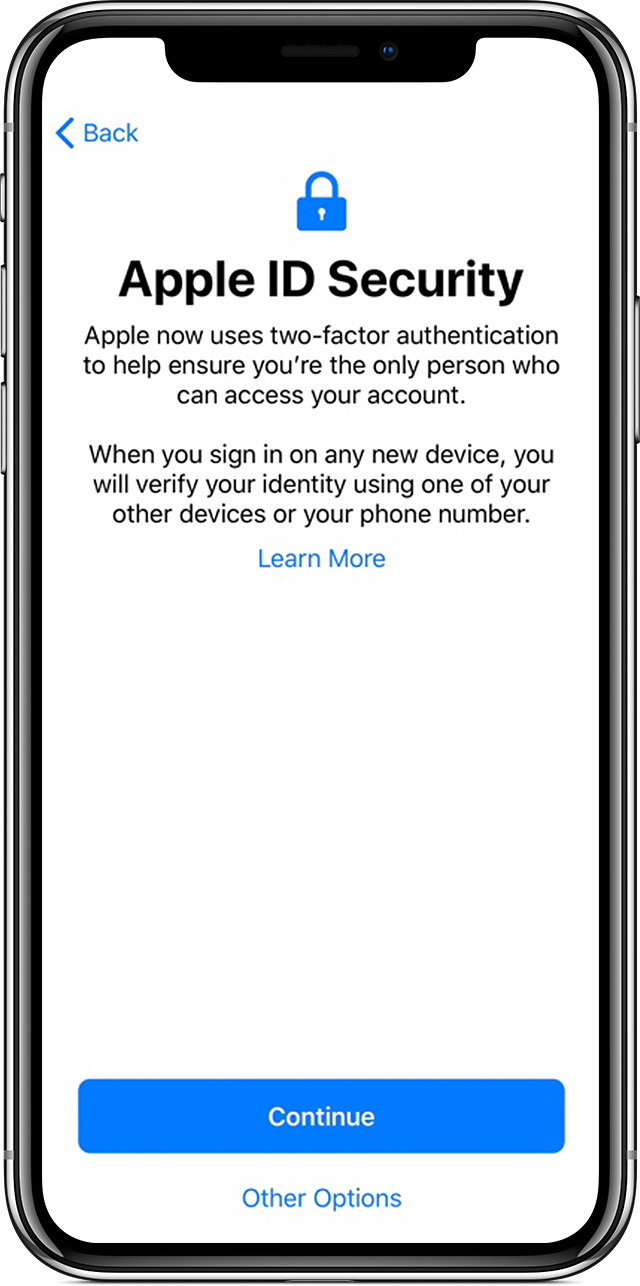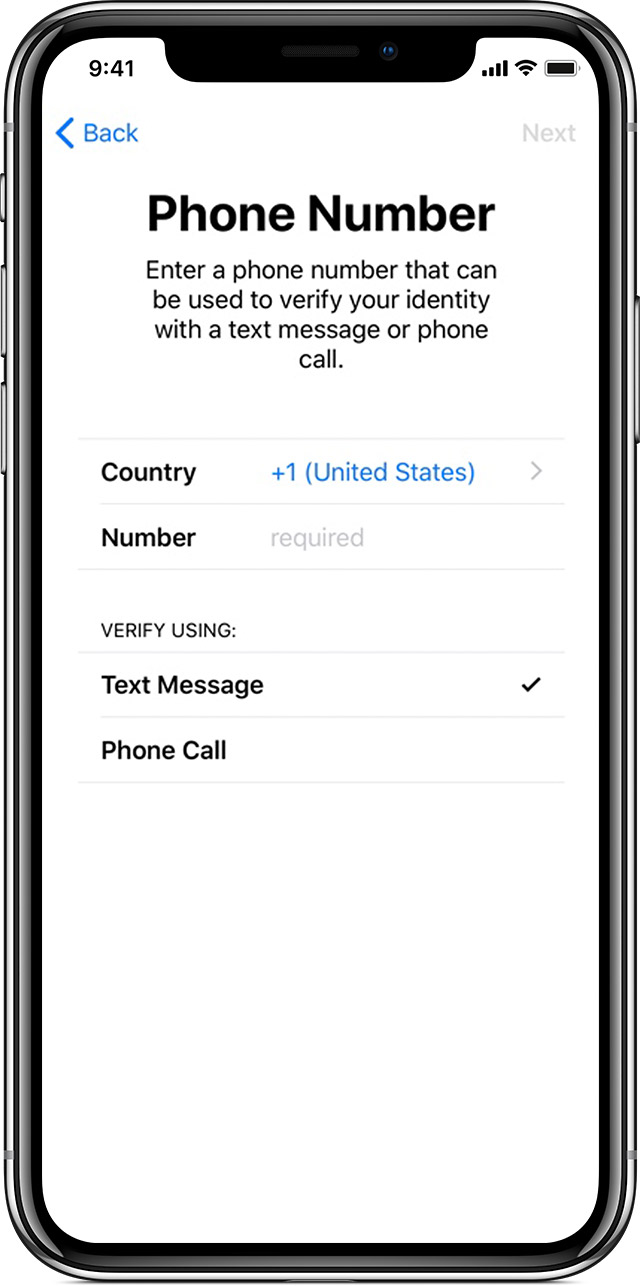Apple ID 双重认证适用情况

谁可以开启双重认证？

• 设有密码且装有 iOS 9 和更高版本的 iPhone、iPad 或 iPod touch
• 装有 OS X El Capitan 和 iTunes 12.3 或更高版本的 Mac
• 装有 watchOS 2 或更高版本的 Apple Watch
• 装有 Apple TVOS 的 Apple TV HD
• 装有 Windows 版 iCloud 和 iTunes 12.3.3 或更高版本的 Windows PC

开启 Apple ID 的双重认证

从新设备上进行设置从已在使用的设备上进行设置

1. 前往“设置”>“[您的姓名]”>“密码与安全性”。如果您使用的是 iOS 10.2 或更低版本，请前往“设置”>“iCloud”。轻点您的 Apple ID >“密码与安全性”。
2. 轻点“打开双重认证”。
3. 轻点“继续”。

1. 更新到最新的 iOS。
2. 在 App Store 或 iTunes Store 中，轻点或右上角您的照片。
3. 轻点“创建新 Apple ID”。
4. 按照屏幕上的指示操作。

1. 前往苹果 () 菜单 >“系统偏好设置”>“iCloud”>“帐户详细信息”。
2. 点按“安全性”。
3. 在屏幕底部，查找标有“打开双重认证”的按钮。

• 阿富汗
• 阿尔及利亚
• 安哥拉
• 亚美尼亚
• 阿塞拜疆
• 巴林
• 孟加拉国
• 贝宁
• 博茨瓦纳
• 布基纳法索
• 布隆迪
• 喀麦隆
• 佛得角
• 中非共和国
• 乍得
• 科特迪瓦
• 科摩罗
• 刚果民主共和国
• 吉布提
• 埃及
• 埃塞俄比亚
• 赤道几内亚
• 厄立特里亚
• 加蓬

• 冈比亚
• 加纳
• 几内亚
• 几内亚比绍
• 印度
• 伊拉克
• 以色列
• 约旦
• 肯尼亚
• 科威特
• 黎巴嫩
• 莱索托
• 利比里亚
• 利比亚
• 马达加斯加
• 马拉维
• 马里
• 毛里塔尼亚
• 毛里求斯
• 摩洛哥
• 莫桑比克
• 纳米比亚
• 尼日尔
• 尼日利亚
• 阿曼
• 巴勒斯坦地区
• 卡塔尔
• 留尼汪
• 刚果共和国
• 卢旺达
• 沙特阿拉伯
• 塞内加尔
• 塞舌尔
• 塞拉利昂
• 索马里
• 南非
• 斯威士兰
• 塔吉克斯坦
• 坦桑尼亚
• 多哥
• 突尼斯
• 土库曼斯坦
• 乌干达
• 阿拉伯联合酋长国
• 西撒哈拉
• 也门
• 赞比亚
• 津巴布韦

• 美属萨摩亚
• 澳大利亚
• 不丹
• 文莱
• 柬埔寨
• 中国大陆 大陆
• 圣诞岛
• 库克群岛
• 斐济
• 法属波利尼西亚
• 关岛
• 香港
• 印度尼西亚
• 日本
• 哈萨克斯坦
• 基里巴斯

• 吉尔吉斯斯坦
• 老挝
• 澳门
• 马来西亚
• 马绍尔群岛
• 蒙古
• 缅甸
• 瑙鲁
• 尼泊尔
• 新喀里多尼亚
• 纽埃
• 新西兰
• 北马里亚纳群岛
• 巴基斯坦
• 帕劳
• 巴布亚新几内亚
• 菲律宾
• 萨摩亚
• 新加坡
• 所罗门群岛
• 韩国
• 斯里兰卡
• 台湾
• 泰国
• 东帝汶
• 托克劳
• 汤加
• 图瓦卢
• 乌兹别克斯坦
• 瓦努阿图
• 越南
• 瓦利斯和富图纳群岛

• 阿尔巴尼亚
• 安道尔
• 奥地利
• 白俄罗斯
• 比利时
• 波斯尼亚和黑塞哥维那
• 保加利亚
• 英属印度洋领地
• 克罗地亚
• 塞浦路斯
• 捷克共和国
• 丹麦
• 爱沙尼亚
• 法罗群岛
• 芬兰
• 法国
• Georgia
• 德国
• 直布罗陀

• 希腊
• 格陵兰
• 格恩西
• 匈牙利
• 冰岛
• 意大利
• 爱尔兰
• 马恩岛
• 泽西岛
• 科索沃
• 拉脱维亚
• 列支敦士登
• 立陶宛
• 卢森堡
• 北马其顿
• 马尔代夫
• 马耳他
• 摩尔多瓦
• 摩纳哥
• 黑山
• 荷兰
• 荷属安的列斯
• 挪威
• 皮特凯恩群岛
• 波兰
• 葡萄牙
• 罗马尼亚
• 俄罗斯
• 圣赫勒拿岛
• 圣马力诺
• 塞尔维亚
• 斯洛伐克
• 斯洛文尼亚
• 西班牙
• 斯瓦巴和扬马延
• 瑞典
• 瑞士
• 土耳其
• 乌克兰
• 英国

• 安圭拉
• 安提瓜和巴布达
• 阿根廷
• 阿鲁巴
• 巴哈马
• 巴巴多斯
• 伯利兹
• 百慕大
• 玻利维亚
• 巴西
• 英属维尔京群岛
• 开曼群岛
• 智利
• 哥伦比亚
• 哥斯达黎加

• 多米尼克
• 多米尼加共和国
• 厄瓜多尔
• 萨尔瓦多
• 格林纳达
• 瓜德罗普
• 危地马拉
• 圭亚那
• 海地
• 洪都拉斯
• 牙买加
• 马提尼克
• 墨西哥
• 尼加拉瓜
• 巴拿马
• 巴拉圭
• 秘鲁
• 法属圣马丁岛
• 荷属圣马丁岛
• 圣基茨
• 圣卢西亚
• 圣文森特和格林纳丁斯
• 苏里南
• 特立尼达和多巴哥
• 特克斯和凯科斯群岛
• 乌拉圭
• 美属维尔京群岛
• 委内瑞拉

• 加拿大
• 波多黎各
• 圣皮埃尔和密克隆群岛
• 美国